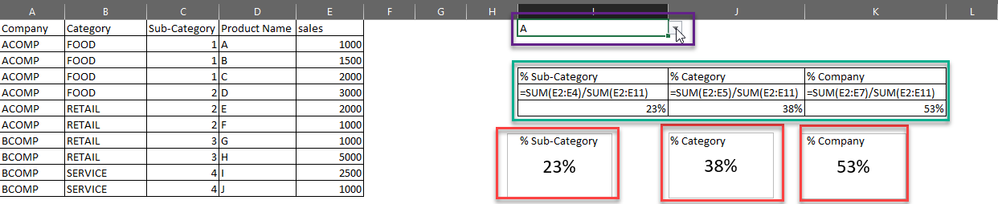cancel
Showing results for
Did you mean:

Fabric is Generally Available. Browse Fabric Presentations. Work towards your Fabric certification with the Cloud Skills Challenge.Frequent Visitor

## SUM By GROUP by Slicer

Hi Guys, I have data like this:

 Company Category Sub-Category Product Name sales ACOMP FOOD 1 A 1000 ACOMP FOOD 1 B 1500 ACOMP FOOD 1 C 2000 ACOMP FOOD 2 D 3000 ACOMP RETAIL 2 E 2000 ACOMP RETAIL 2 F 1000 BCOMP RETAIL 3 G 1000 BCOMP RETAIL 3 H 5000 BCOMP SERVICE 4 I 2500 BCOMP SERVICE 4 J 1000

The case when I put the slicer based on Product name, the value also keep SUM of Product Name.

My Expectation result, when I put the slicer based on Product Name can show SUM of Group in a Card Visual, looks like:Sorry for my explanation in Excel mode, that's to make it clear enough.

Does someone know how to this calculation in Dax

BR

1 ACCEPTED SOLUTIONCommunity Support

Hi @tian

I think you need to create an unrelated Product Name table. Then build a slicer by this table and create measures.

``Product Name = VALUES('Table'[Product Name])``

Measure codes:

``````% Sub-Category =
VAR _SelectProductName =
SELECTEDVALUE ( 'Product Name'[Product Name] )
VAR _SubCategory =
CALCULATE (
MAX ( 'Table'[Sub-Category] ),
FILTER ( ALL ( 'Table' ), 'Table'[Product Name] = _SelectProductName )
)
VAR _SalesbyGroup =
CALCULATE (
SUM ( 'Table'[sales] ),
FILTER ( ALL ( 'Table' ), 'Table'[Sub-Category] = _SubCategory )
)
VAR _Total =
CALCULATE ( SUM ( 'Table'[sales] ), ALL ( 'Table' ) )
RETURN
DIVIDE ( _SalesbyGroup, _Total )``````
``````% Category =
VAR _SelectProductName =
SELECTEDVALUE ( 'Product Name'[Product Name] )
VAR _Category =
CALCULATE (
MAX ( 'Table'[Category] ),
FILTER ( ALL ( 'Table' ), 'Table'[Product Name] = _SelectProductName )
)
VAR _SalesbyGroup =
CALCULATE (
SUM ( 'Table'[sales] ),
FILTER ( ALL ( 'Table' ), 'Table'[Category] = _Category )
)
VAR _Total =
CALCULATE ( SUM ( 'Table'[sales] ), ALL ( 'Table' ) )
RETURN
DIVIDE ( _SalesbyGroup, _Total )``````
``````% Company =
VAR _SelectProductName =
SELECTEDVALUE ( 'Product Name'[Product Name] )
VAR _Company =
CALCULATE (
MAX ( 'Table'[Company] ),
FILTER ( ALL ( 'Table' ), 'Table'[Product Name] = _SelectProductName )
)
VAR _SalesbyGroup =
CALCULATE (
SUM ( 'Table'[sales] ),
FILTER ( ALL ( 'Table' ), 'Table'[Company] = _Company )
)
VAR _Total =
CALCULATE ( SUM ( 'Table'[sales] ), ALL ( 'Table' ) )
RETURN
DIVIDE ( _SalesbyGroup, _Total )``````

Result is as below.Best Regards,
Rico Zhou

If this post helps, then please consider Accept it as the solution to help the other members find it more quickly.

2 REPLIES 2Community Support

Hi @tian

I think you need to create an unrelated Product Name table. Then build a slicer by this table and create measures.

``Product Name = VALUES('Table'[Product Name])``

Measure codes:

``````% Sub-Category =
VAR _SelectProductName =
SELECTEDVALUE ( 'Product Name'[Product Name] )
VAR _SubCategory =
CALCULATE (
MAX ( 'Table'[Sub-Category] ),
FILTER ( ALL ( 'Table' ), 'Table'[Product Name] = _SelectProductName )
)
VAR _SalesbyGroup =
CALCULATE (
SUM ( 'Table'[sales] ),
FILTER ( ALL ( 'Table' ), 'Table'[Sub-Category] = _SubCategory )
)
VAR _Total =
CALCULATE ( SUM ( 'Table'[sales] ), ALL ( 'Table' ) )
RETURN
DIVIDE ( _SalesbyGroup, _Total )``````
``````% Category =
VAR _SelectProductName =
SELECTEDVALUE ( 'Product Name'[Product Name] )
VAR _Category =
CALCULATE (
MAX ( 'Table'[Category] ),
FILTER ( ALL ( 'Table' ), 'Table'[Product Name] = _SelectProductName )
)
VAR _SalesbyGroup =
CALCULATE (
SUM ( 'Table'[sales] ),
FILTER ( ALL ( 'Table' ), 'Table'[Category] = _Category )
)
VAR _Total =
CALCULATE ( SUM ( 'Table'[sales] ), ALL ( 'Table' ) )
RETURN
DIVIDE ( _SalesbyGroup, _Total )``````
``````% Company =
VAR _SelectProductName =
SELECTEDVALUE ( 'Product Name'[Product Name] )
VAR _Company =
CALCULATE (
MAX ( 'Table'[Company] ),
FILTER ( ALL ( 'Table' ), 'Table'[Product Name] = _SelectProductName )
)
VAR _SalesbyGroup =
CALCULATE (
SUM ( 'Table'[sales] ),
FILTER ( ALL ( 'Table' ), 'Table'[Company] = _Company )
)
VAR _Total =
CALCULATE ( SUM ( 'Table'[sales] ), ALL ( 'Table' ) )
RETURN
DIVIDE ( _SalesbyGroup, _Total )``````

Result is as below.Best Regards,
Rico Zhou

If this post helps, then please consider Accept it as the solution to help the other members find it more quickly.Super User

@tian , based on what I got

measure = divide(sum(Table[Sales]), calculate(sum(Table[Sales]), all(Table)))Announcements#### Power BI Monthly Update - November 2023

Check out the November 2023 Power BI update to learn about new features.#### Fabric Community News unified experience

Read the latest Fabric Community announcements, including updates on Power BI, Synapse, Data Factory and Data Activator.#### The largest Power BI and Fabric virtual conference

130+ sessions, 130+ speakers, Product managers, MVPs, and experts. All about Power BI and Fabric. Attend online or watch the recordings.Top Solution Authors
Top Kudoed Authors
Users online (2,128)